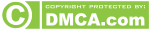# Difference between Resultant and Equilibrants

Following are four main difference in Resultant and Equilibrants force

No. Resultant Equilibrant
1. Definition: A resultant of number of forces acting on a body is a single. Force which can produce the same effect on the body as it is produced by all the forces acting together. Definition: An equilibrant of number of forces acting on a body is a single force which cancels the effect of resultant of a system of forces or which brings the system and the body is equilibrium.
2. Resultant (net) force causes the displacement of a body (i.e. body moves). Equilibrant keeps the body at rest (i.e. in equilibrium).
3. The set of forces which causes the displacement of a body are called as component of resultant or component forces. The set of forces which keeps the body at restate known as equilibrium forces or components of equilibrant.
4. R represents the resultant of two concurrent forces P & Q. E represents the equilibrant of two concurrent forces P & Q.